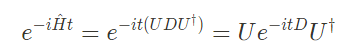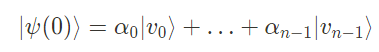# Exact Diagonalization Put Briefly.

## On key tools for simulating Hamiltonians

TL;DR Exact diagonalization is a common numerical technique employed to simulate Hamiltonians. It leverages the well-known linear algebraic process of diagonalization — whereby you factorize the Hamiltonian into its action with respect to its eigenbasis (finding all eigen[states/values] en route). Read on to find out how.

The overarching objective is to compute the unitary operator corresponding to the time evolution of a given initial state (this is what it means to simulate a Hamiltonian). By Schrödinger’s equation, we understand that this unitary operator is in fact the matrix exponential of the Hamiltonian multiplied by a couple of scalar factors including time:

So computationally, this method answers the question: how do we obtain the unitary operator characterized by `exp(-iHt)` ?

Suppose we’re given a Hermitian matrix, `A`. By the Spectral Theorem, we know that all normal matrices are diagonalizable, and inheriting from that all Hermitian matrices — being normal — are diagonalizable too. Further, they’re unitarily diagonalizable, a property arising from the self-adjointness of `A`. So we can write `A = UDU^†` where `D` is diagonal and `U`is some unitary operator. To then compute `exp(A)`, we have that

Thus, in order to exponentiate any Hermitian matrix we “just” need to diagonalize it first. This trivially generalizes to computing `exp(-iHt)` as wellIf you’re not convinced, expand these steps with the power series representation

Recall that the exponential of a diagonal matrix is simply the exponential of each of its’ diagonal elements. Alas, evolving our Hamiltonian reduces to diagonalizing the matrix.

But take caution, despite its’ mild appearance, diagonalizing the Hamiltonian involves computing all of its’ eigenstates and eigenvalues — a task that falls prey to the catastrophic dimension growth inherent to quantum mechanics. There is no free lunch! The exponential growth in the dimensionality of the Hilbert space prevents this technique from being used to simulate systems of larger than approximately 20 qubits. Yet here is an interesting approximation technique to simulate systems larger in size.

Though it may not provide much practical value beyond a system size of 20 qubits, it remains an important technique to consider.

# A superficially different approach

I encountered the following approach when reading through S. Aaronson’s lecture notes and wanted to show how it’s practically equivalent to the approach we just discussed.

Say we apply the unitary operator `exp(-iHt)`to the eigenstate `|v_j⟩` . Since eigenstates of a Hermitian matrix are retained through matrix exponentiation, we arrive at

Meaning that the initial state (an energy eigenstate) has merely picked up a global phase proportional to the eigenvalue. Now, recall that all Hermitian matrices — of which Hamiltonians fall under — share the property that their eigenstates collectively form an orthonormal basis spanning the entire Hilbert space. Thus any arbitrary state `|Ψ⟩` can be decomposed as|v_i⟩ are the energy eigenstates, and α_i the accompanying complex amplitudes

From this perspective, evolving a system under a Hamiltonian for a time `t`is equivalent to doing

So computing `exp(-iHt)|Ψ_0⟩` (our core objective) can be done by representing `|Ψ_0⟩` with respect to the eigenbasis followed by tacking on relative phases of each constituent eigenstate, equal to `λt`.

So how does this line up with our prior approach? Here we calculate `exp(-iHt)` on the operand vector directly. In contrast, exact diagonalization requires that we compute the isolated unitary operator `exp(-iHt)` first, followed by its application onto `|Ψ_0⟩` .

However, to employ this fact still requires you to find each eigenstate and eigenvalue (akin to diagonalizing), so it’s of no practical difference which method you undertake!

# Summary

Exact diagonalization can be summarized in the following simple process:

If you enjoyed this brief note, or spotted a mistake, feel free to reach me here.

--

--